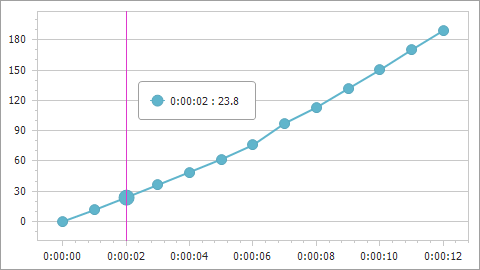21.2 (EAP/Beta)
21.1
20.2
20.1
19.2
The page you are viewing does not exist in version 19.2. This link will take you to the root page.
19.1
The page you are viewing does not exist in version 19.1. This link will take you to the root page.
18.2
The page you are viewing does not exist in version 18.2. This link will take you to the root page.
18.1
The page you are viewing does not exist in version 18.1. This link will take you to the root page.
17.2
The page you are viewing does not exist in version 17.2. This link will take you to the root page.

# ChartCalculatedField.FieldType Property

Gets or sets the type of calculated field values.

Namespace: DevExpress.XtraCharts

Assembly: DevExpress.XtraCharts.v21.1.dll

## Declaration

``````[XtraChartsLocalizableCategory(XtraChartsCategory.Data)]
public ChartCalculatedFieldType FieldType { get; set; }``````

## Property Value

Type Description
ChartCalculatedFieldType

The type of resulted values.

Available values:

Show 12 items
Name Description
None

Indicates that the field’s type is undefined and determined based on the returned object during chart initialization.

String

Indicates that the field returns a string value as a sequence of UTF-16 code units (the String type).

DateTime

Indicates that the field returns a value expressed as a date and time of day (the DateTime type).

TimeSpan

Indicates that the field returns a value as a time interval (the TimeSpan type).

Byte

Indicates that the field returns an 8-bit unsigned integer value (the Byte type).

Int16

Indicates that the field returns a 16-bit signed integer value (the Int16 type).

Int32

Indicates that the field returns a 32-bit signed integer value (the Int32 type).

Float

Indicates that the field returns a single-precision floating-point value (the Single type).

Double

Indicates that the field returns a double-precision floating-point value (the Double type).

Decimal

Indicates that the field returns a decimal value (the Decimal type).

Boolean

Indicates that the field returns a Boolean (true or false) value (the Boolean type).

Guid

Indicates that the field returns a global unique identifier value (the Guid type).

## Remarks

The FieldType property specifies the type of values (Boolean, DateTime, String, and etc.) a calculated field returns after its expression is evaluated. The ChartCalculatedFieldType enumeration lists the available types.

## Example

The following example shows how to create a calculated field and then use this field as a data source for a series. The field’s values are calculated by the following expression: [Time.Seconds] * [Velocity].``````using DevExpress.XtraCharts;
using System;
using System.Collections.Generic;
using System.Windows.Forms;

namespace CalculatedFieldExample {
public partial class Form1 : Form {
//...
private void Form1_Load(object sender, EventArgs e) {
chartControl1.DataSource = GetDataPoints();

ChartCalculatedField calcField = new ChartCalculatedField();
calcField.Expression = "[Time.Seconds] * [Velocity]";
calcField.FieldType = ChartCalculatedFieldType.Double;
calcField.Name = "Displacement";

Series series = new Series("series", ViewType.Line);
series.ArgumentDataMember = "Time";
XYDiagram diagram = chartControl1.Diagram as XYDiagram;
diagram.AxisX.TimeSpanScaleOptions.MeasureUnit = TimeSpanMeasureUnit.Second;
diagram.AxisY.WholeRange.AlwaysShowZeroLevel = false;
}
public List<DataPoint> GetDataPoints() {
List<DataPoint> dataPoints = new List<DataPoint>() {
new DataPoint (new TimeSpan(0, 0, 0), 10),
new DataPoint (new TimeSpan(0, 0, 1), 11.46),
new DataPoint (new TimeSpan(0, 0, 2), 11.90),
//...
// Other data points here.
// ...
new DataPoint (new TimeSpan(0, 0, 12), 15.756)
};
return dataPoints;
}
}
public class DataPoint {
public TimeSpan Time { get; set; }
public double Velocity { get; set; }
public DataPoint(TimeSpan time, double velocity) {
this.Time = time;
this.Velocity = velocity;
}
}
}
``````